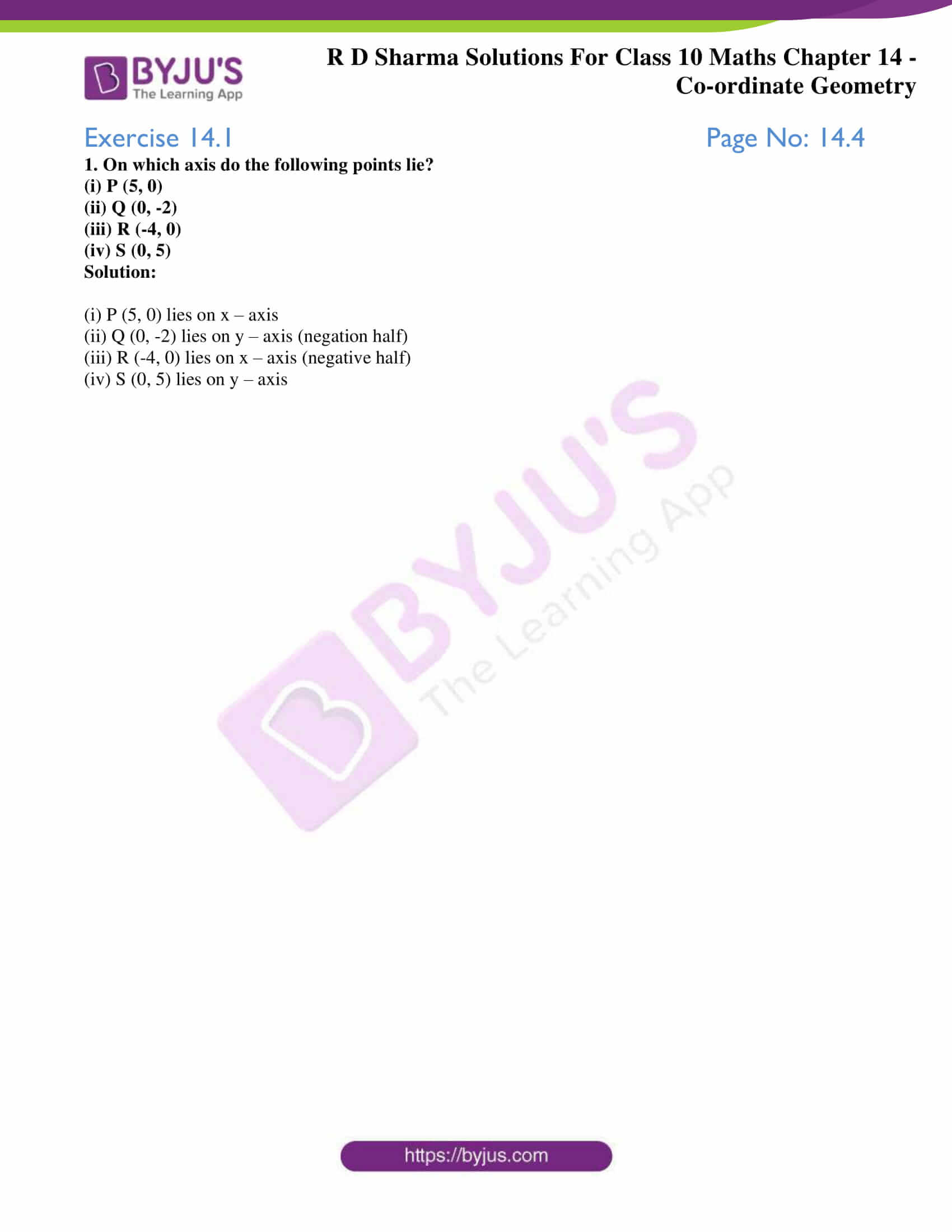# RD Sharma Solutions for Class 10 Maths Chapter 14 Co-ordinate Geometry Exercise 14.1

For locating a point on a plane, we need a pair of mutually perpendicular lines known as coordinate axes. Introduction to these rectangular coordinate axes, cartesian coordinates of a point, quadrants are the key things tested in this exercise. The RD Sharma Solutions Class 10 is available for students to clarify any doubts pertaining to this chapter. Students can also download the RD Sharma Solutions for Class 10 Maths Chapter 14 Co-ordinate Geometry Exercise 14.1 PDF provided below.

## RD Sharma Solutions for Class 10 Maths Chapter 14 Co-ordinate Geometry Exercise 14.1 Download PDF### Access RD Sharma Solutions for Class 10 Maths Chapter 14 Co-ordinate Geometry Exercise 14.1

1. On which axis do the following points lie?

(i) P (5, 0)

(ii) Q (0, -2)

(iii) R (-4, 0)

(iv) S (0, 5)

Solution:

(i) P (5, 0) lies on x – axis

(ii) Q (0, -2) lies on y – axis (negation half)

(iii) R (-4, 0) lies on x – axis (negative half)

(iv) S (0, 5) lies on y – axis